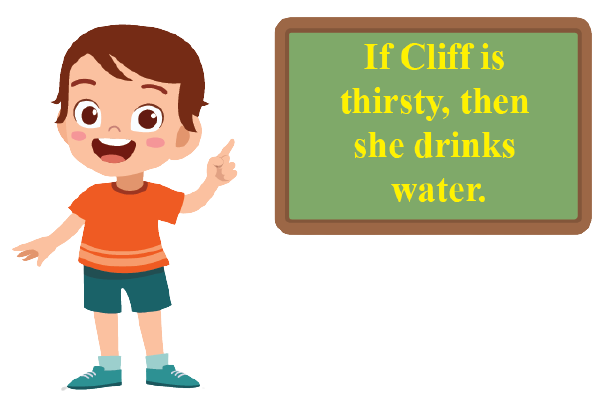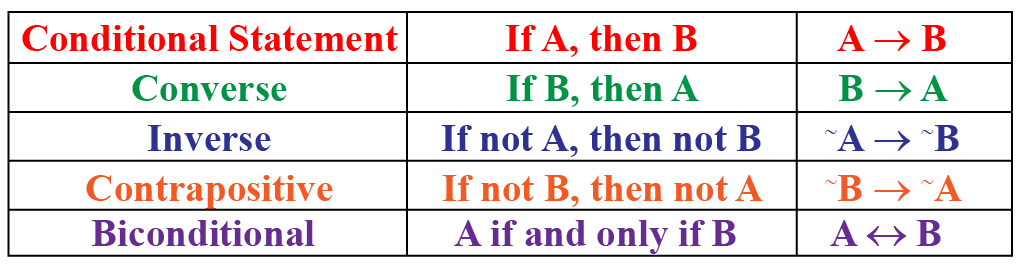# Conditional Statement

Conditional Statement

A conditional statement is a part of mathematical reasoning which is a critical skill that enables students to analyze a given hypothesis without any reference to a particular context or meaning. In layman words, when a scientific inquiry or statement is examined, the reasoning is not based on an individual's opinion. Derivations and proofs need a factual and scientific basis.

Mathematical critical thinking and logical reasoning are important skills that are required to solve maths reasoning questions.In this mini-lesson, we will explore the world of conditional statements. We will walk through the answers to the questions like what is meant by a conditional statement, what are the parts of a conditional statement, and how to create conditional statements along with solved examples and interactive questions.

## Lesson Plan

 1 What Is Meant By a Conditional Statement? 2 Tips and Tricks 3 Solved Examples 4 Interactive Questions 5 Challenging Question

## What Is Meant By a Conditional Statement?

A statement that is of the form "If p, then q" is a conditional statement. Here 'p' refers to 'hypothesis' and 'q' refers to 'conclusion'.

For example, "If Cliff is thirsty, then she drinks water."This is a conditional statement. It is also called an implication.

'$$\rightarrow$$' is the symbol used to represent the relation between two statements. For example, A$$\rightarrow$$B. It is known as the logical connector. It can be read as A implies B.

Here are two more conditional statement examples

Example 1: If a number is divisible by 4, then it is divisible by 2.

Example 2: If today is Monday, then yesterday was Sunday.

## What Are the Parts of a Conditional Statement?

Hypothesis (if) and Conclusion (then) are the two main parts that form a conditional statement.

Let us consider the above-stated example to understand the parts of a conditional statement.

Conditional Statement: If today is Monday, then yesterday was Sunday.

Hypothesis: "If today is Monday."

Conclusion: "Then yesterday was Sunday."

On interchanging the form of statement the relationship gets changed.

To check whether the statement is true or false here, we have subsequent parts of a conditional statement. They are:

• Converse
• Inverse
• Contrapositive
• Biconditional Statement

Let us consider hypothesis as statement A and Conclusion as statement B.### Converse of Statement

When hypothesis and conclusion are switched or interchanged, it is termed as converse statement.
For example,

Conditional Statement: “If today is Monday, then yesterday was Sunday.”

Hypothesis: “If today is Monday”

Converse: “If yesterday was Sunday, then today is Monday.”

Here the conditional statement logic is, If B, then A (B → A)

### Inverse of Statement

When both the hypothesis and conclusion of the conditional statement are negative, it is termed as an inverse of the statement.
For example,

Conditional Statement:“If today is Monday, then yesterday was Sunday”.

Inverse: “If today is not Monday, then yesterday was not Sunday.”

Here the conditional statement logic is, If not A, then not B (~A → ~B)

### Contrapositive Statement

When the hypothesis and conclusion are negative and simultaneously interchanged, then the statement is contrapositive.
For example,

Contrapositive: “If yesterday was not Sunday, then today is not Monday”

Here the conditional statement logic is, if not B, then not A (~B → ~A)

### Biconditional Statement

The statement is a biconditional statement when a statement satisfies both the conditions as true, being conditional and converse at the same time.
For example,

Biconditional: “Today is Monday if and only if yesterday was Sunday.”

Here the conditional statement logic is, A if and only if B (A ↔ B)

## How to Create Conditional Statements?

Here, the point to be kept in mind is that the 'If' and 'then' part must be true.

If a number is a perfect square, then it is even.

• 'If' part is a number that is a perfect square.

Think of 4 which is a perfect square.

This has become true.

• The 'then' part is that the number should be even. 4 is even.

This has also become true.

Thus, we have set up a conditional statement.

Let us hypothetically consider two statements, statement A and statement B. Observe the truth table for the statements:

 Statement A Statement B A → B Truth Truth Truth Truth False False False Truth Truth False False Truth

According to the table, only if the hypothesis (A) is true and the conclusion (B) is false then, A → B will be false, or else A → B will be true for all other conditions.Tips and Tricks

• A sentence needs to be either true or false, but not both, to be considered as a mathematically accepted statement.
• Any sentence which is either imperative or interrogative or exclamatory cannot be considered a mathematically validated statement.
• A sentence containing one or many variables is termed as an open statement. An open statement can become a statement if the variables present in the sentence are replaced by definite values.

## Solved Examples

Let us have a look at a few solved examples on conditional statements.

 Example 1

Identify the types of conditional statements.

Solution

There are four types of conditional statements:

• If condition
• If-else condition
• Nested if-else
 Example 2

Ray tells "If the perimeter of a rectangle is 14, then its area is 10."

Which of the following could be the counterexamples? Justify your decision.

a) A rectangle with sides measuring 2 and 5

b) A rectangle with sides measuring 10 and 1

c) A rectangle with sides measuring 1 and 5

d) A rectangle with sides measuring 4 and 3

Solution

a) Rectangle with sides 2 and 5: Perimeter = 14 and area = 10

Both 'if' and 'then' are true.

b) Rectangle with sides 10 and 1: Perimeter = 22 and area = 10

'If' is false and 'then' is true.

c) Rectangle with sides 1 and 5: Perimeter = 12 and area = 5

Both 'if' and 'then' are false.

d) Rectangle with sides 4 and 3: Perimeter = 14 and area = 12

'If' is true and 'then' is false.

 Example 3

Joe examined the set of numbers {16, 27, 24} to check if they are the multiples of 3. He claimed that they are divisible by 9. Do you agree or disagree? Justify your answer.

Solution

Conditional statement: If a number is a multiple of 3, then it is divisible by 9.

Let us find whether the conditions are true or false.

a) 16 is not a multiple of 3. Thus, the condition is false.

16 is not divisible by 9. Thus, the conclusion is false.

b) 27 is a multiple of 3. Thus, the condition is true.

27 is divisible by 9. Thus, the conclusion is true.

c) 24 is a multiple of 3. Thus the condition is true.

24 is not divisible by 9. Thus the conclusion is false.

 Example 4

Write the converse, inverse, and contrapositive statement for the following conditional statement.

If you study well, then you will pass the exam.

Solution

The given statement is - If you study well, then you will pass the exam.

It is of the form, "If p, then q"

The converse statement is, "You will pass the exam if you study well" (if q, then p).

The inverse statement is, "If you do not study well then you will not pass the exam" (if not p, then not q).

The contrapositive statement is, "If you did not pass the exam, then you did not study well" (if not q, then not p).

## Interactive Questions

Here are a few activities for you to practice. Select/Type your answer and click the "Check Answer" button to see the result.Challenging Question
1. Choose a number. Double it. Add 6. Double again. Subtract 4. Divide by 4. Subtract 2.
a) Make a conjecture about the relation between the number you picked and the final result.
b) Can you find the converse, inverse, biconditional, and a contrapositive statement for the same?
2. If 2a + 3 <  10, then a = 3. Write the converse, inverse, and contrapositive statements and verify their truthfulness.

## Let's Summarize

The mini-lesson targeted the fascinating concept of the conditional statement. The math journey around conditional statements started with what a student already knew and went on to creatively crafting a fresh concept in the young minds. Done in a way that not only it is relatable and easy to grasp, but also will stay with them forever.

At Cuemath, our team of math experts is dedicated to making learning fun for our favorite readers, the students!

Through an interactive and engaging learning-teaching-learning approach, the teachers explore all angles of a topic.

Be it worksheets, online classes, doubt sessions, or any other form of relation, it’s the logical thinking and smart learning approach that we, at Cuemath, believe in.

## FAQs on Conditional Statement

### 1. What is the most common conditional statement?

'If and then' is the most commonly used conditional statement.

### 2. When do you use a conditional statement?

Conditional statements are used to justify the given condition or two statements as true or false.

### 3. What is if and if-else statement?

If is used when a specified condition is true.
If-else is used when a particular specified condition is not satisfying and is false.

### 4. What is the symbol for a conditional statement?

'$$\rightarrow$$' is the symbol used to represent the relation between two statements. For example, A$$\rightarrow$$B. It is known as the logical connector. It can be read as A implies B.

### 5. What is the Contrapositive of a conditional statement?

When the hypothesis and conclusion are negative and simultaneously interchanged, then the statement is contrapositive.
For example,

Contrapositive: “If yesterday was not Sunday, then today is not Monday”

If not B, then not A (~B → ~A)

### 6. What is a universal conditional statement?

Conditional statements are those statements where a hypothesis is followed by a conclusion. It is also known as an " If-then" statement. If the hypothesis is true and the conclusion is false, then the conditional statement is false. Likewise, if the hypothesis is false the whole statement is false. Conditional statements are also termed as implications.

Conditional Statement: If today is Monday, then yesterday was Sunday

Hypothesis: "If today is Monday."

Conclusion: "Then yesterday was Sunday."

If A, then B (A → B)

More Important Topics
More Important Topics
Learn from the best math teachers and top your exams

• Live one on one classroom and doubt clearing
• Practice worksheets in and after class for conceptual clarity
• Personalized curriculum to keep up with school